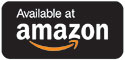Catalog Higher Ed Mathematics Elementary Algebra

# Elementary Algebra

## by Terentie Bortis

Third Edition

### Print Version

#### Paperback Price: \$50.71

ISBN: 978-1-50669-547-1

542 pages

Suggested retail price shown above.The primary goal of this textbook is to provide students and instructors with an introductory algebra textbook that is straightforward and provides the simplest and shortest explanation for each mathematical concept included in the book. To help make this book usable in a class lecture format, each topic is first defined in plain and concise language, and built on using numerous examples that increase in difficulty from simple to more challenging. Each section ends with more than enough exercises for a fifty minutes lecture and homework assignment.

Terentie Bortis has taught math for over 30 years, most recently at Long Beach City College.

CHAPTER 1: Fundamental Concepts

CHAPTER 2: Equations and Inequalities in One Variable

CHAPTER 3: Graphing Linear Equations and Inequalities in Two Variables

CHAPTER 4: Systems of Linear Equations and Inequalities

CHAPTER 5: Exponents and Polynomials

CHAPTER 6: Factoring Polynomials

CHAPTER 7: Rational Expressions and Equations

CHAPTER 8: Radical Expressions and Equations